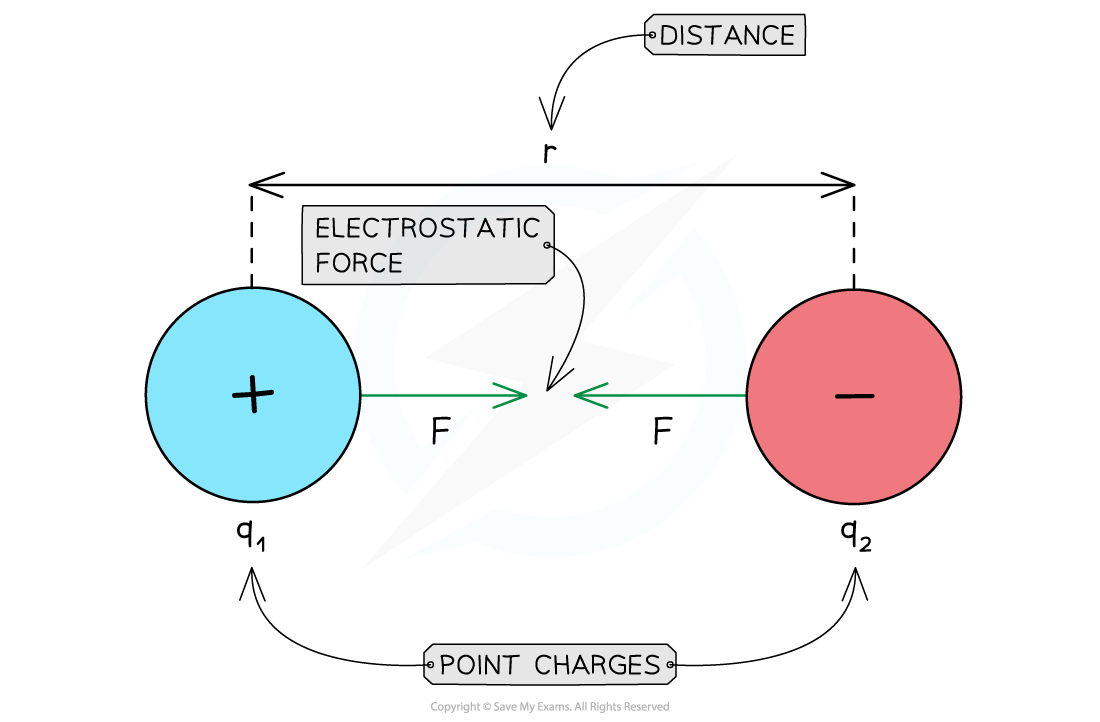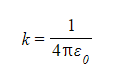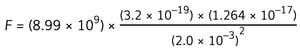# IB DP Physics: SL复习笔记5.1.5 Coulomb’s Law

### Coulomb's Law

• Any charged particle generates an electric field
• This field exerts a force on any other charged particle within range
• The electrostatic force between two charges is defined by Coulomb’s Law, which states that:

The attractive or repulsive electrostatic force between two point charges is directly proportional to the product of the charges and inversely proportional to the square of their separation

• According to Coulomb's law, the electrostatic force between two point charges is calculated as follows:• Where:
• F = electrostatic force (N)
• q1, q2 = magnitudes of the charges (C)
• r = distance between the centres of the two charges (m)
• k = Coulomb constant (N m2 C–2)Attractive electrostatic force between two opposite charges

• Coulomb's constant is given by:• The value of k depends on the material between the charges
• In a vacuum, k = 8.99 × 109 N m2 C–2
• ε0 is the permittivity of free space
• ε0 = 8.85 × 10–12 C2 N–1 m–2 and refers to charges in a vacuum
• The value of the permittivity of air is taken to be the same as ε0
• Any other material has a higher permittivity ε > ε0
• ε is a measure of the resistance offered by a material in creating an electric field within it

#### Repulsive & Attractive Forces

• For like charges:
• The product q1q2 is positive
• F is positive
• The charges repel each other
• For opposite charges:
• The product q1q2 is negative
• F is negative
• The charges attract each other

#### Worked Example

An alpha particle is situated 2.0 mm away from a gold nucleus in a vacuum.Assuming them to be point charges, calculate the magnitude of the electrostatic force acting on each of the charges.

• Atomic number of helium = 2
• Atomic number of gold = 79

Step 1: Write down the known quantities

• Distance, r = 2.0 mm = 2.0 × 10–3 m
• Elementary charge, e = 1.60 × 10–19 C (from the data booklet)

Note that you must convert the distance from millimetres (mm) into metres (m)

Step 2: Calculate the charges of the alpha particle and gold nucleus

• The elementary charge e (when taken to be positive) is the charge of a proton
• An alpha particle (helium nucleus) has 2 protons
• So its charge is:

q1 = 2 × (1.60 × 10–19) = 3.2 × 10–19 C

• A gold nucleus has 79 protons
• So its charge is:

q2 = 79 × (1.60 × 10–19) = 1.264 × 10–17 C

Step 3: Write down the equation to calculate the electrostatic forceStep 4: Substitute the numbers into the equationF = 9.1 × 10–21 N

#### Exam Tip

You do not need to memorise the numerical value of the Coulomb's constant k or that of the permittivity of free space ε0. They will both be given in the data booklet.

Unless specified in the question, you should assume that charges are located in a vacuum.

You should note that Coulomb's law can only be applied to charged spheres whose size is much smaller than their separation. Only in this case, the point charge approximation is valid. You must remember that the separation r must be taken from the centres of the spheres.

You cannot use Coulomb's law to calculate the electrostatic force between charges distributed on irregularly-shaped objects.